Related Articles
• Explore Our Geeks Community

# Problems on Motional EMF

Electromagnetic or magnetic induction is the generation of an electromotive force across an electrical conductor in a changing magnetic field. Michael Faraday discovered induction in 1831, and James Clerk Maxwell mathematically defined it as Faraday’s law of induction. Lenz’s law describes the direction of the induced field. The Maxwell–Faraday equation, one of Maxwell’s four electromagnetic equations, was derived from Faraday’s law.

Electromagnetic induction has helped electrical components such as inductors and transformers, as well as devices such as electric motors and generators.

### Motional EMF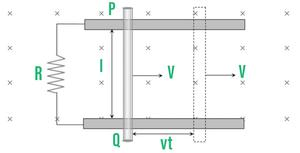The concept of the generated area can also be utilized to explain the occurrence of induced emf when a conductor moves between two parallel conductor rails (The area swept by the conductor in the magnetic field, during its motion).

In time t distance traveled by conductor d = vt.

Area generated, A = l vt

Flux linked with this area, ϕ = BA = Blvt

Hence, the induced emf,

|e| = dϕ/dt = Bvl

Induced current,

I = e/R

and

I = Bvl/R

### Fleming’s Right-Hand Rule

According to this law, if we stretch the right-hand thumb and two nearby fingers perpendicular to one another, the central finger will point in the direction of the induced current, with the first finger-pointing in the direction of the magnetic field and the thumb pointing in the direction of conductor motion.

### Magnetic Force on a Conductor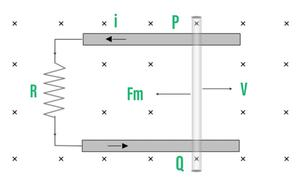The circuit now has current flowing across it (conductor). A current-carrying conductor experiences a force Fm=Bil (maximum) when moving in a magnetic field, the direction of which may be determined using Fleming’s left-hand rule.

As a result, the magnetic force Fm=Bil acting on conductor PQ is directed in the opposite direction of its velocity, and Fm=Bil=B(Bvl/R)l ; Fm=B2vl2/R

### Power Dissipated in Moving the Conductor

For the uniform motion of the rod PQ, the rate of completing mechanical work by an external agent or mechanical power provided by an external source is given as:

Pmech=pext=dW/dt=Fext.v=B2vl2/R× v

⇒Pmech=B2V2l2/R

In addition, the electrical power dissipated in resistance is used to calculate the rate of heat dissipation across the resistance.

Pthermal=H/t=i2R=(Bvl/R)2.R

PthermalB2v2l2/R

### Motion of Conductor Rod in a Vertical Plane

When a conducting rod is released from rest (at t=0), its speed (v) increases, resulting in an induced emf (e), induced current I, and magnetic force (Fm), while the weight of the rod remains constant.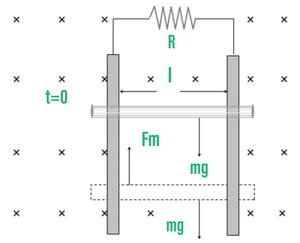Rod will achieve a constant maximum (terminal) velocity vr if Fm=mg

So B2Vr2l2/R=mg

⇒vr=mgR/B2l2

### Motion of Conducting Rod on an Inclined Plane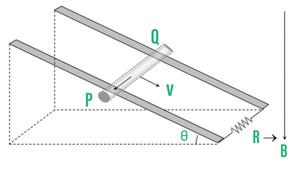When a conductor begins to slide from the top of an inclined plane, it slides perpendicular to its length but at a (90–θ) angle to the direction of a magnetic field, as depicted.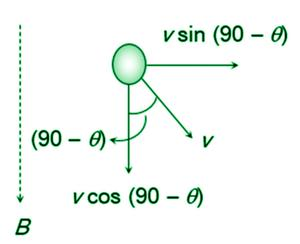Hence induced emf across the ends of a conductor

e=Bvsin(90–θ)l=Bvlcosθ

So induced current i=Bvlcosθ/R (Directed from Q to P).

The rod will move down with constant velocity only if:

Fm cosθ=mg cos(90–θ)=mg sinθ

Bil cosθ=mg sinθ

B (BvTl cosθ/R) l cosθ=mg sinθ

⇒vT=mg R sinθ / B2l2cos2θ

The following are the emfs induced in various examples:

Movement of a train in Earth’s magnetic field: Because the axle of the train’s wheels cuts the vertical component Bv of Earth’s magnetic field, a potential difference between the ends of the axle of the wheels is induced when the train moves on tracks, and the magnetic flux associated with it varies. The potential difference, also known as the emf, is created. e=Bvlv Where l denotes the axle length and v denotes the train’s speed.

The motion of an aeroplane in Earth’s magnetic field: Because the aeroplane cuts the vertical component Bv of Earth’s magnetic field, a potential difference or emf is induced across the wings of an aeroplane flying horizontally at a specific height. As a result, the induced emf is e=Bvlv volt, the length l of an aeroplane’s wings, and the speed v of the flight.

Orbital satellite: There will be no emf induced if the orbital plane of an artificial satellite with a metallic surface corresponds with the equatorial plane of the Earth. When the orbital plane intersects the equatorial plane, emf is produced.

### Motional EMF Due to Rotational Motion

• Conducting Rod: A conducting rod of length l is rotated along an axis passing through its fixed end and perpendicular to its length with constant angular velocity (ω). The magnetic field (B) is perpendicular to the plane of the paper.

Emf induces across the ends of the rod e=1/2 Bl2ω=Bl2πv=Bl2π/T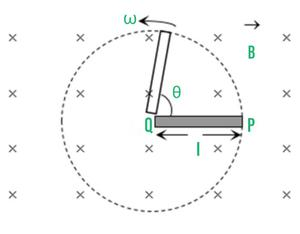• Cycle Wheel: In a given magnetic field, each spoke of a conducting wheel rotates with angular velocity.

Each metal spoke becomes an identical cell of emf e (say) due to flux cutting, and all such identical cells are connected in a parallel way enet=e (emf of a single cell). Assume N is the number of spokes.

enet=1/2Bωl2 ; ω=2πv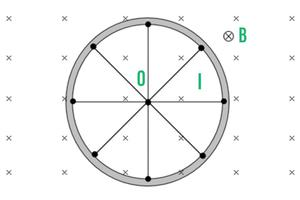• Faraday Copper Disc Generator: The spinning action of the disc chops away at the magnetic field lines.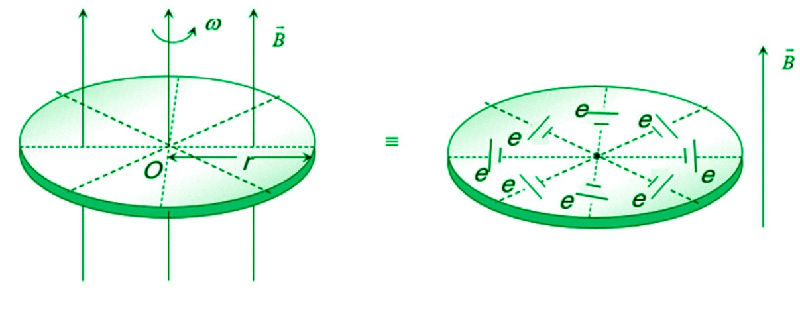Uncountable radial conductors can be considered to make up a metal disc. These radial conductors cutaway magnetic field lines when the metal disc rotates in the transverse magnetic field. Because of this flux cutting, all become identical cells each of emf ‘e′ where e=1/2Bωr2 ,

Because all identical cells are connected in parallel, the net emf for the disc is zero.

enet=e=1/2Bωr2=B(πr2)v

• Semicircular Conducting Loop: The loop plane is in the plane of the paper for the given figure, a semicircular conducting loop (ACD) of radius ‘r′ with centre at O. The loop is now rotated at a constant angular velocity(ω) along an axis that passes through O and is perpendicular to the paper’s plane. The loop’s effective resistance is R.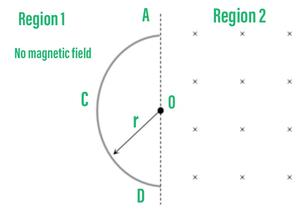In time t the area swept by the loop in the field, i.e. region II

A=1/2r(rθ)=1/2r2ωt ; dA/dt =r2ω/2

Flux link with the rotating loop at a time t,
ϕ=BA

Hence induced emf in the loop in magnitude |e|=dϕ/dt=B dA/dt=Bωr2/2 and induced current  i=|e|/R=Bωr2/2R

### Periodic EMI

Assume that a rectangular coil with N turns is first put in a magnetic field that is perpendicular to its plane.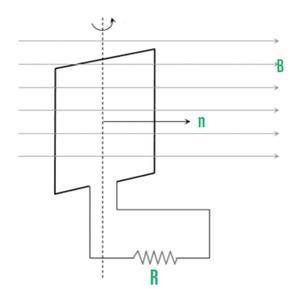ω–Angular speed

V–Frequency of rotation of the coil

R–Resistance of coil

For uniform rotational motion with ω, the flux linked with the coil at any time

ϕ=NBAcosθ=NBAcosωt(asθ=ωt)

ϕ=ϕ0cosωt where ϕ=NBA=flux amplitude or maximum flux

Induced EMF in the Coil

e=–dϕ/dt=NBAω sinωt⇒e=e0sinωt

Where

e0=emf amplitude or max.

emf=NBAω=ϕ0ω

Induced Current

At any time t , i=e/R=e0Rsinωt=i0 sinωt

where i0=current amplitude or max. current i0=e0R=NBAωR=ϕ0ωR

### Sample Problems

Problem 1: A circular antenna with a surface size of 3 m2 has been built in Madurai. The plane of the antenna’s area is inclined at 47o to the Earth’s magnetic field direction. Find the magnetic flux associated with the antenna if the magnitude of the Earth’s field at that location is 40773.9 nT.

Solution

B = 40773.9 nT ;  θ = 90º  – 47º  = 43°;

A = 3m2

We know that  ΦB = BAcosθ

= 40773.9 × 10-9 × 3 × cos43º

=89.47 × 10-6 Wb

=89.47 μ Wb

Problem 2: In a homogeneous magnetic field of 0.2 T, a circular loop with an area of 5 x 10-2m2 revolves. If the loop revolves around its perpendicular to the magnetic field diameter. When the loop’s plane is I normal to the field, (ii) inclined 60o to the field, and (iii) parallel to the field, find the magnetic flux associated with it.

Solution

A = 5 ´ 10-2 m2; B = 0.2 T

(i) θ = 0°

ΦB =BA cosθ

= 0.2 × 5 × 10-2 × cos 0°

=10-2 Wb

(ii) θ=30°

ΦB=BA cosθ

=0.2 × 5 × 10-2 × cos 30°

=8.66 × 10-3 Wb

(iii) θ=90°

=0

Problem 3: A coil has 1000 turns and a surface area of 500 cm2. A magnetic induction field of 2 × 10-5 Wb/m2 is placed at right angles to the coil’s plane. In 0.2 seconds, the coil is rotated through 180 degrees. The coil’s average induced emf (in millivolts)

Solution

emf= △t/△ϕ

​= 2ϕ/t

= 2×B×A×n/t

​= 2 × 2 × 10−5 × 500 ×10−4 × 1000/ 0.2

=10×10−3

V=10 mV

Problem 4: Calculate the motional emf induced along a 20.0 km conductor moving at an orbital speed of 7.80 km/s perpendicular to Earth’s 5.00×10−5T magnetic field.

Solution

We know

ε=Bℓv

ε=Bℓv

=(5.00×10−5T)(2.00×104m)(7.80×103m/s)

=7.80×103V.

Problem 5: In a magnetic field with a flux density of 2 Wb m-2, a closed coil with 40 turns and an area of 200 cm2 is turned. It spins in 0.2 seconds from a position where its plane forms a 30o angle with the field to a location perpendicular to the field. Determine the magnitude of the induced emf in the coil as a result of its rotation.

Solution

N = 40 turns; B = 2 Wb m-2

A = 200 cm2 = 200 ´ 10-4 m2 ;

Initial flux, Φi = BA cos θ

=2 × 200 × 10-4 × cos60°

=2 × 10-2 Wb

Final flux, Φf =BA cos θ

=2 × 200 × 10-4 × cos0°

=4 × 10-2 Wb

Magnitude of the induced emf is

= 40 × (4 × 10-2 – 2 × 10-2) / 0.2

= 4V

Problem 6: The magnetic flux is driven into the paper perpendicular to the plane of the circuit. If the magnetic flux varies over time according to the following equation: When t = 3 s and B = (2t3 +3t2 + 8t +5) mWb, what is the magnitude of the induced emf in the loop? Determine the current flow direction in the circuit.

Solution

(i)ΦB = (2t 3 +3t2 + 8t +5) mWb;

N =1, t = 3 s

ε=d(NΦB)/dt

=(6t2 + 6t + 8) × 10-3

At t=3s

ε=[(6×9)+(6×3)+8] × 10-3

=80 mV

(ii) The magnetic flux associated with the loop grows with time. The direction of the induced current should be such that it opposes the flux increase, according to Lenz’s law. As a result, the induced current flows in such a way that it creates a magnetic field that is the polar opposite of the provided field. This magnetic field is pointing outwards perpendicularly. As a result, the induced current flows in the opposite direction.

Problem 7: A metal conductor of length 1 m rotates vertically about one of its ends at angular velocity 5 radians per second. If the horizontal component of earth’s magnetic field is 0.2×10 −4 T, then the e.m.f developed between the two ends of the conductor?

Solution

B=0.2×10−4 T

ε= Bωl/2

= 0.2×10−4×5×1/2

​=50μV

Whether you're preparing for your first job interview or aiming to upskill in this ever-evolving tech landscape, GeeksforGeeks Courses are your key to success. We provide top-quality content at affordable prices, all geared towards accelerating your growth in a time-bound manner. Join the millions we've already empowered, and we're here to do the same for you. Don't miss out - check it out now!

Previous
Next